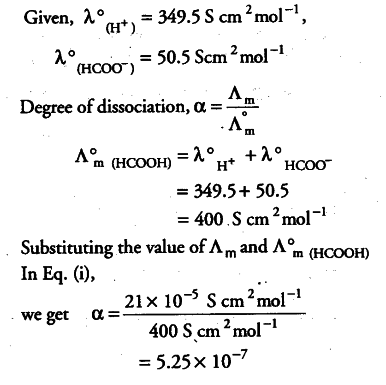# Conductivity of 2.5 x \${{10}^{-4}}\$ M methanoic acid

Conductivity of 2.5 x \${{10}^{-4}}\$ M methanoic acid is 5.25 x \${{10}^{-5}}\$ S \${{cm}^{-1}}\$. Calculate its molar conductivity and degree of dissociation.

Conductivity of methanoic acid = 5.25 x {{10}^{-5}} S {{cm}^{-1}}

Concentration of methanoic acid = 2.5 X {{10}^{-4}} M = 2.5 x {{10}^{-4}} x 1000 mol {{cm}^{-3}} = 0.25 mol {{cm}^{-3}}

Molar conductivity,

A_{ m } = K/C = 5.25 x {{10}^{-5}} S {{cm}^{-1}} / 0.25 mol {{cm}^{-3}}

= 21 x {{10}^{-5}} S {{cm}^{2}} {{10}^{-5}} S {{mol}^{-1}}